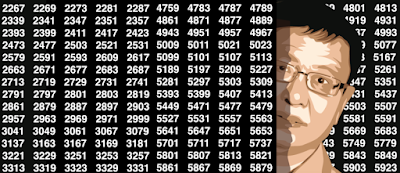## Sunday, September 24, 2017

### Interesting Application of the Greedy Algorithm for Egyptian Fractions

Here we discuss a new system to represent numbers, for instance constants such as Pi, e, or log 2, using rational fractions. Each iteration doubles the precision (the number of correct decimals computed) making it converging much faster than current systems such as continued fractions, to represent any positive real number. The algorithm discussed here is equivalent to the greedy algorithm to compute Egyptian fractions, except that we use it here mostly for irrational numbers.
For any real number x, the representation is as follows:
where the integer numbers p(k) are computed iteratively with the following two-steps iteration, starting with k = 1::
where the brackets represent the floor function which always returns an integer. You start with a seed p(1) = 1 (though you can work with other seed values) and loop over k = 1, 2, and so on. As k tends to infinity, x(k) tends to x. The speed of convergence is remarkable, yielding 14 decimals after five or six iterations, and doubling the precision at each iteration, because p(k+1) is of the same order of magnitude as the square of p(k). It works well if x is between 1 and 2,
Examples
Here are a few interesting examples:
• For x = p - 2, we get p(1) = 1, p(2) = 8, p(3) =  61, p(4) = 5020, p(5) = 128541459, yielding x(5) = 1.14159265358979, with 13 correct decimals.
• For x = 1 + log 2, we get p(1) = 1, p(2) = 2, p(3) =  6, p(4) = 38, p(5) = 6071, p(6) = 144715222, yielding x(6) = 1.69314718055995, with 14 correct decimals.
• For x = 2, we get p(1) = 1, p(2) = 2, p(3) =  3, p(4) = 7, p(5) = 43, p(6) = 1807, yielding x(6) = 1.99999969357507, with 6 correct decimals.
The sequence p(1), p(2), and so on uniquely characterizes the number x. Note that for k as small as 6 or 7, p(k) becomes so large that you need to use special arithmetic (long numbers) to perform the computations in any programming language, as you have reached the limit in terms of computer precision.
By contrast, continued fractions converge much more slowly, click here to see the difference. The 6-th convergent for p (with continued fractions) is 104348 / 33215 = 3.141592654. Compare this with our approximation x(6) for p-2, which has a far higher precision.
General framework and proof of convergence
This methodology generalizes as follows: Start with a value p(1) with x > 1 / p(1), and iteratively compute p(k+1) as the smallest number from an infinite set E, such that x(k+1)   <  x, with
In our particular case, E is the set of all positive integers, and p(1) = 1. With p(1) = 1, convergence is fastest when x gets very close (above) 1. If x = 1 and p(1) = 2, then p(k+1) = 1 + p(k) * (p(k) - 1), explaining the incredibly fast convergence: This case is identical to the case x = 2 and p(1) = 1, discussed in the above section.  The numbers p(k) produced in this case, namely 2, 3, 7, 43, 1807 and so on, correspond to Sylvester's sequence
Note that E could be any set of strictly positive numbers (for instance, prime numbers, or numbers that are not even integers), as long of the sum of the inverse of the elements of E, is infinite.
Unlike with other number representations, all numbers seem to be created equal here, whether they are a fraction of two integers, irrational or transcendental. Note that irrational numbers will always satisfy the fact that x(k) is different from x, guaranteeing convergence. With other number representations (continued fractions or base 10 representation), some classes of numbers result in periodicity.
The coefficients p(k) could be used to build random number generators. Finally, a similar methodology can be developed to represent numbers as infinite products, click here for details
For a simple recursion yielding all the binary digits of the square root of 2, one at a time, click here. For another interesting challenge, read the section "Potential Areas of Research" in my article How to detect if numbers are random or not. For other articles featuring difficult mathematical problems, click here. For a statistical problem with several potential applications (clustering, data reduction) click here and read the last section. More challenges can be found here. .